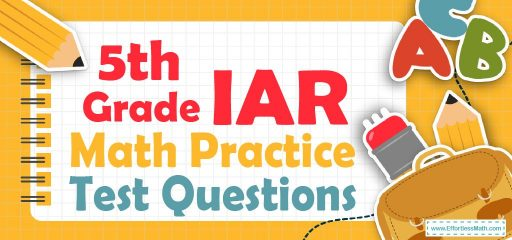# 5th Grade IAR Math Practice Test QuestionsThe practice resources that 5th-grade students use to prepare for the Illinois Assessment of Readiness (IAR) Math Test should be of standard quality, credibility, and content so that the student is not misled.

Therefore, in this article, we have compiled the 5th-Grade IAR Math Practice Test Questions along with a step-by-step guide to solving them.

These 5th-Grade IAR Math Practice Test Questions cover the most important math concepts of the IAR Math Test and get students to a high level of readiness.

The Absolute Best Book to Ace the 5th Grade IAR Math Test

## 10 Sample 5th Grade IAR Math Practice Questions

1- What is the volume of this box?

A. $$24 \space cm^3$$

B. $$32 \space cm^3$$

C. $$162 \space cm^3$$

D. $$192\space cm^3$$

2- How many square feet of tile is needed for $$18$$ feet to $$18$$ feet room?

A. $$72$$ Square Feet

B. $$108$$ Square Feet

C. $$216$$ Square Feet

D. $$324$$ Square Feet

3- Of the $$2,400$$ videos available for rent at a certain video store, $$600$$ are comedies. What percent of the videos are comedies?

A. $$18 ½ \%$$

B. $$20 \%$$

C. $$22 \%$$

D. $$25 \%$$

4- How many $$3 × 3$$ squares can fit inside a rectangle with a height of $$54$$ and width of $$12$$?

A. $$72$$

B. $$62$$

C. $$50$$

D. $$44$$

5- ABC Corporation earned only $$200,000$$ during the previous year, two-thirds only of the management’s predicted income. How much earnings did the management predict?

A. $$20,000$$

B. $$30,000$$

C. $$300,000$$

D. $$340,000$$

6- William keeps track of the length of each fish that he catches. Following are the lengths in inches of the fish that he caught one day:$$13, 14, 9, 11, 9, 10, 18$$
What is the median fish length that William caught that day?

A. $$11$$ Inches

B. $$9$$ Inches

C. $$12$$ Inches

D. $$13$$ Inches

7- $$9 + [8 × 5] ÷ 2 =$$ ? __________

8- What is the median of these numbers? $$4, 9, 13, 8, 15, 18, 5$$

A. $$8$$

B. $$9$$

C. $$13$$

D. $$15$$

9-The area of the base of the following cylinder is $$50$$ square inches and its height is $$10$$ inches. What is the volume of the cylinder? __________

10- A rope $$13$$ yards long is cut into $$4$$ equal parts. Which expression does NOT equal the length of each part?

A. $$13 ÷ 4$$

B. $$\frac{13}{4}$$

C. $$4 ÷ 13$$

D. $$4\sqrt{13}$$

Best 5th Grade IAR Math Prep Resource

1- D
Use the volume of the cube formula.
$$Voluem= length × width × height ⇒ V= 6 × 4 × 8 ⇒ V=192 \space cm^3$$

2- D
Find the area of the room which is a square. Use the area of square formula.
$$S= a^2 ⇒ S= 18$$ feet $$× 18$$ feet $$= 324$$ square feet

3- D
Use the percent formula:
$$part =\frac{percent}{100}× whole$$
$$600 =\frac{percent}{100}× 2400⇒ 600= percent × 24 ⇒percent=25$$

4- A
Use the area of the rectangle formula.
$$A= a × b ⇒ A= 54 × 12 ⇒ A= 648$$
Divide the area by $$9$$ ($$3 × 3 = 9$$ squares) to find the number of squares needed. $$648 ÷ 9 = 72$$

5- C
ABC Corporation’s income = $$\frac{2}{3}$$ management’s predicted income.
$$200,000 = \frac{2}{3}$$ management’s predicted income
management’s predicted income $$= 200,000×\frac{3}{2} =300,000$$

6- A
Write the numbers in order:
$$9, 9, 10, 11, 13, 14, 18$$
The Median is the number in the middle. Therefore, the median is $$11$$.

7- 29
Use PEMDAS (order of operation):
$$9 + [8 × 5] ÷ 2 = 9 + (40) ÷ 2 = 9 + (40 ÷ 2) = 29$$

8- B
Write the numbers in order:
$$4, 5, 8, 9, 13, 15, 18$$
The Median is the number in the middle. Therefore, the median is $$9$$.

9- 500
Use the volume of cylinder formula.
$$Voluem= base × height ⇒ V=50 × 10 ⇒ V=500$$

10- C
$$13$$ yards long rope is cut into $$4$$ equal parts. Therefore, $$13$$ should be divided by $$4$$. Only option C is NOT $$13$$ divided by $$4$$. (It is $$4$$ divided by $$13$$)

The Best Books to Ace the 5th Grade IAR Math Test

### What people say about "5th Grade IAR Math Practice Test Questions - Effortless Math: We Help Students Learn to LOVE Mathematics"?

No one replied yet.

X
27% OFF

Limited time only!

Save Over 27%

SAVE $5 It was$18.57 now it is \$13.57